### Home > PC > Chapter 9 > Lesson 9.3.2 > Problem9-116

9-116.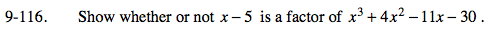Set up the generic rectangle with the divisor on the left of the box. Input only the first terms of the dividend.

Since x divides into x³, x² times, write the x² on top and multiply x² with the −5 and fill in the grid with the product.

Add an expression to −5x² in order to get the needed 4x². Add it to the right of x³.

Dividing x into 9x² gives 9x. Add the 9x at the top. Then multiply 9x and −5 and fill the answer in the grid.

Add an expression to −45x in order to get the needed −11x. Add it to the right of 9x².

Dividing x into 34x gives 34. Add the 34 at the top. Then multiply 34 and −5 and fill the answer in the grid.

Add an expression to −170 in order to get the needed −30. Add it to the right of 34x. This is the remainder. Add it to the top as a ratio.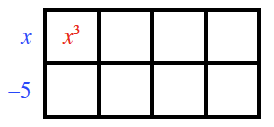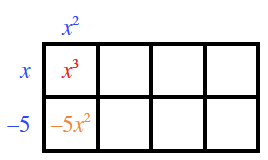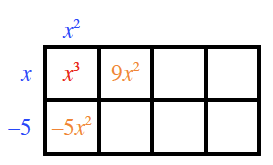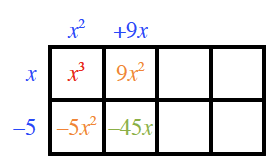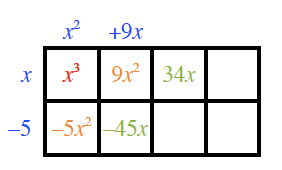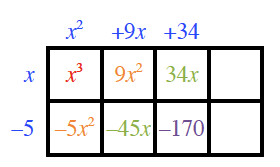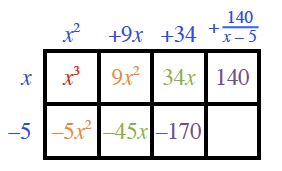Since there is a remainder, x − 5 is not a factor!